Advances in Difference Equations

, 2019:413

Numerical solutions of nonlinear fractional model arising in the appearance of the strip patterns in two-dimensional systems

Open Access
Research

Abstract

The main aim of this paper is to present a comparative study of modified analytical technique based on auxiliary parameters and residual power series method (RPSM) for Newell–Whitehead–Segel (NWS) equations of arbitrary order. The NWS equation is well defined and a famous nonlinear physical model, which is characterized by the presence of the strip patterns in two-dimensional systems and application in many areas such as mechanics, chemistry, and bioengineering. In this paper, we implement a modified analytical method based on auxiliary parameters and residual power series techniques to obtain quick and accurate solutions of the time-fractional NWS equations. Comparison of the obtained solutions with the present solutions reveal that both powerful analytical techniques are productive, fruitful, and adequate in solving any kind of nonlinear partial differential equations arising in several physical phenomena. We addressed $$L_{2}$$ and $$L_{\infty }$$ norms in both cases. Through error analysis and numerical simulation, we have compared approximate solutions obtained by two present aforesaid methods and noted excellent agreement. In this study, we use the fractional operators in Caputo sense.

Keywords

Homotopy analysis transform method (HATM) Homotopy polynomial (HP) Newell–Whitehead–Segal (NWS) equation Residual power series method

26A33 465M22

1 Introduction

Differential equations of nonlinear nature are a practically very useful device for recitation of different physical phenomena, particularly when it is fractional in character. In support of illustration, these equations are gradually more applied to the problems related to diverse branches of engineering [1, 2, 3, 4]. An enormous attempt has been taken throughout the previously years to get healthy and proficient arithmetical and logical methods for solving nonlinear fractional differential equations (FDEs) [5, 6, 7, 8, 9, 10, 11, 12, 13, 14]. This work emphasized that the NSW equation is taken to find the solutions using aforesaid methods. The modified homotopy analysis transform method (MHATM) method is a combination of the Laplace transform and homotopy analysis methods with HP [15, 16, 17, 18]. The RPSM is constructed from the generalized Taylor series, which is a prevailing technique for solving nonlinear FDEs [19, 20, 21, 22, 23, 24]. The advantage of the RPSM method is that it is not affected by computational round-off errors and also does not require large computer memory and extensive time. Moreover, this method computes the coefficients of the power series by a chain of equations with one or more variables, which indicates a better convergence of the RPSM.

Recently, the NWS equation gained more attention because NWS plays a vital part in nonlinear systems. The NWS equation describes the appearance of the stripe pattern in two-dimensional systems. Moreover, as this is an important model in the field of fluid dynamics, it has numerous applications in fluid dynamics such as traveling wave patterns in binary fluids. A new approach using ADM to evaluate the numerical solution of TFNWS is mentioned in . In  the authors analyzed the fractional NWS equation for Riemann fractional space–time, space, and time derivatives. Two methods, Laplace decomposition and finite difference, to solve the numerical approximation of NWS equations are given in . Investigations related to the mathematical biological model in connection with the NWS equation are presented by Korkmaz . Approximate solutions of the NWS using a new iterative method are given in . In  the authors gave a comparative study on the reduced transform method and ADM insight of NWS equation, and in  a combined form of ADM and Elzaki method is used to solve the NWS equations. Numerous papers studied the solutions of NWS equation by applying different approaches. In  the tanh function technique is used to get the exact solution of generalized NWS, and in  the HPM is used to solve the nonlinear NWS differential equations. To approximate the solutions of NWS equation from fluid mechanics, Macías-Díaz and Ruiz-Ramírez  proposed a method called the finite-difference method. The class of NWS equations with Lie and “nonclassical” symmetry points of view was studied in . Some papers used the variational iteration method (VIM) or modified VIM to solve the NWS equations [36, 37]. Graham  studied the two-dimensional NWS equations (also see . Kumar and Sharma  combined the HAM and Sumudu transform (ST) to solve the NWS equation. The linear and nonlinear NWS equations are evaluated in  with the help of HPM and a hybrid of the Fourier transform and ADM. The NWS amplitude equation and algebraic traveling wave NWS equation were studied in  and , respectively. For more detail on fractional-order NWS equations in diverse points of view, we refer the interesting readers to the recent papers [44, 45].

We consider the fractional model of NWS equation  in the operator form
$$D^{\lambda }_{t} \xi (\eta , t) = k D ^{2}_{\eta } \xi (\eta , t) + a\xi (\eta ,t)- b \xi ^{c}(\eta , t),\quad 0< \lambda \leq 1,$$
(1.1)
with initial condition
$$\xi (\eta ,0)= f(\eta ),$$
(1.2)
where c is positive integer, k, a, and $$b \in \mathbb{R}$$ (real numbers) with $$k>0$$, and $$D^{\lambda }$$ is the Caputo derivative of order λ. The first term $$D=\frac{\partial \xi }{\partial \eta }$$ represents the variation of $$\xi (\eta ,t)$$ with time and fixed location. The term $$D^{2}=\frac{\partial ^{2} \xi }{\partial \eta ^{2}}$$ denotes the variation with variable η at a particular time, and the remaining term $$a\xi - b \xi ^{c}$$ signifies the effect of the source term. Various methods are applied to solve different types of NWS equations in physics [31, 46, 47, 48, 49].

Theorem 1.1

Letfbe a function represented by a fractional power series (FPS) at$$t=t_{0}$$
$$\sum_{k=0}^{\infty }\sum _{l=0}^{m-1} d_{kl}(\eta ) (t-t_{0})^{k\lambda + l},\quad 0\leq m-1< \lambda \leq m, t_{0} \leq t < t_{0}+\mathbb{R}.$$
If$$D^{k\lambda + l} f(t)$$are continuous on$$(t_{0},t_{0}+\mathbb{R})$$, $$k=0,1,2,\ldots$$ , then the coefficients$$d_{kl}$$are given by
$$d_{kl}=\frac{D^{k\lambda + l} f(t_{0})}{\varGamma (k \lambda + l+1)},\quad k=0,1,2,\ldots,$$
where$$D^{k \lambda } = D^{\lambda }$$, $$D^{\lambda },\ldots,D^{\lambda }$$ (ktimes), and the radius of convergence is$$\mathbb{R}$$.

2 Basic idea of MHATM

2.1 The analytical procedure

The fundamental scheme of the MHATM is illustrated by taking common appearance of FDEs:
$$D^{\lambda }_{t} \xi (\eta ,t)+ K[\eta ] \xi (\eta ,t)+M[\eta ] \xi (\eta ,t)= Q(\eta ,t), \quad t>0, \eta \in \mathbb{R}, 0< \lambda \leq 1,$$
(2.1)
where $$K[\eta ]$$ and $$M[\eta ]$$ are linear and nonlinear terms, respectively, and $$Q(\eta ,t)$$ and $$\xi (\eta ,t)$$ are continuous and unknown functions, respectively. For clearness, we neglect all conditions.
Now the methodology consists of first applying the Laplace transform to both sides of equation (2.1):
$$L\bigl[D^{\lambda }_{t} \xi (\eta ,t)+ K[ \eta ] \xi (\eta ,t)+M[\eta ] \xi (\eta ,t)\bigr]= L\bigl[Q(\eta ,t)\bigr].$$
(2.2)
Next, using the differentiation property of the Laplace transform, we have
$$L\bigl[\xi (\eta ,t)\bigr]-\frac{1}{s^{\lambda }}\sum _{k=0}^{n-1} s^{\lambda -k-1}\xi ^{k}(\eta ,0)+\frac{1}{s^{ \lambda }} L \bigl(K[\eta ] \xi (\eta ,t)+M[\eta ] \xi (\eta ,t)- Q( \eta ,t) \bigr)=0.$$
(2.3)
We define the nonlinear operator
\begin{aligned}[b] N\bigl[\aleph (r,t;z)\bigr]&= L\bigl[\aleph (\eta ,t;z)\bigr]-\frac{1}{s^{\lambda }} \sum_{k=0}^{n-1} s^{\lambda -k-1}\xi ^{k}(\eta ,0)\\ &\quad {}+\frac{1}{s^{\lambda }}L \bigl(K[\eta ] \xi (\eta ,t)+M[\eta ] \xi (\eta ,t)- Q(\eta ,t) \bigr), \end{aligned}
(2.4)
where $$z \in [0,1]$$ is an embedding parameter, and $$\aleph (\eta ,t;z)$$ is a real function of η, t, and z. Generalizing the traditional homotopy analysis methods , we construct the zero-order deformation equation
$$(1-z)L\bigl[\aleph (\eta ,t;z)-\xi _{0}(\eta ,t)\bigr]=\hbar z H(\eta ,t)N\bigl[ \aleph (\eta ,t;z)\bigr],$$
(2.5)
where ħ is a nonzero auxiliary parameter, which helps us to increase the convergence, $$H(\eta ,t)$$ is an auxiliary function, $$\xi _{0}(\eta ,t)$$ is an initial guess of $$\xi (\eta ,t)$$, and $$\aleph (\eta ,t;z)$$ is an unknown function.
Consequently, we obtain the mth-order deformation equation
\begin{aligned} \xi _{m}(\eta ,t) &=(\chi _{m}+ \hbar ) \xi _{m-1}- \hbar (1-\chi _{m}) \sum_{i=0}^{j-1} t^{i} \xi ^{(i-1)}(0) \\ &\quad {}+\hbar L^{-1} \Biggl(\frac{1}{s^{\lambda }} L \Biggl(K_{m-1}[t] \xi _{m-1}(t)+ \sum _{k=0}^{m-1} P_{k}(\xi _{0},\xi _{1},\ldots ,\xi _{m})-Q( \eta ,t) \Biggr) \Biggr), \end{aligned}
(2.6)
where $$P_{k}$$ is the HP given by .
For the expediency point of view, the appearance of nonlinear operator form has been customized in HATM, that is, the nonlinear term $$M[\eta ,t] \xi (\eta ,t)$$ is stretched in the form of HP as
$$M\bigl[\xi (\eta ,t)\bigr]= M \Biggl(\sum_{k=0}^{m-1} \xi _{m}(\eta ,t) \Biggr)= \sum_{m=0}^{\infty }P_{m} \xi ^{m}.$$
(2.7)
The innovation of our planned algorithm is to construct and escalate the nonlinear expression as a sequence of HP in equation (2.6). Next, from equation (2.6) we can compute different values of $$\xi _{m}(\eta ,t)$$ for $$m\geq 1$$. Consequently, we find the whole series solution of equation (2.1) as
$$\xi (\eta ,t)= \xi _{0} (\eta ,t)+ \sum_{m=1}^{\infty } \xi _{m} (\eta ,t).$$
(2.8)

To illustrate the efficiency and accuracy of the MHATM, we consider two examples.

Example 1

Taking the constant values $$k=-1$$, $$a=-2$$, and $$b=0$$ in Eq. (1.1). Therefore Eq. (1.1) is reduced to the linear TFNWS equation 
$$D^{\lambda }_{t} \xi (\eta ,t)= D ^{2}_{\eta } \xi (\eta ,t) - 2 \xi (\eta ,t), \quad 0< \lambda \leq 1,$$
(2.9)
with initial condition $$\xi (\eta ,0)= e^{\eta }$$ and exact solution $$\xi _{\mathrm{exact}}(\eta ,t)=e^{\eta -t}$$, respectively .
Taking the Laplace transform of both sides of equation (2.9), we get
$$s^{\lambda } L\bigl[\xi (\eta ,t)\bigr]-s^{\lambda -1} \xi (\eta ,0)- L \bigl[D^{2} _{\eta } \xi - 2 \xi \bigr]=0.$$
(2.10)
In this case the nonlinear operator defined as
$$N\bigl[\aleph (\eta ,t;z)\bigr]= L \bigl[\aleph (\eta ,t;z)\bigr]- \frac{1}{s} e^{\eta }-s ^{-\lambda } L \bigl[D^{2}_{\eta } \aleph (\eta ,t;z) - 2 \aleph (\eta ,t;z)\bigr].$$
(2.11)
Thus we obtain the mth-order deformation equation
$$L\bigl[\xi (\eta ,t)-\chi _{m} \xi _{m-1}(\eta ,t)\bigr]= \hbar R_{m}(\vec{\eta } _{m-1},\eta ,t).$$
(2.12)
Taking the inverse Laplace transform of both sides in equation (2.12), we get
$$\xi (\eta ,t)=\chi _{m} \xi _{m-1}(\eta ,t) + \hbar L^{-1} \bigl[R_{m}( \vec{\eta }_{m-1},\eta ,t) \bigr],$$
(2.13)
where
\begin{aligned} R_{m}(\vec{\eta }_{m-1},\eta ,t) &= L[\xi _{m-1}]- \frac{1-\chi _{m}}{s} e^{\eta }-s^{-\lambda }L\bigl[D^{2}_{\eta } \xi - 2 \xi \bigr],\quad m \ge 1. \end{aligned}
(2.14)
Now the solution of the mth-order deformation equation is
\begin{aligned} \xi _{m}(\eta ,t) &=(\chi _{m} +\hbar ) \xi _{m-1}(\eta ,t)-\hbar (1-\chi _{m}) e^{\eta }-\hbar L^{-1}\bigl[s^{-\lambda } L\bigl[D^{2}_{\eta } \xi - 2 \xi \bigr]\bigr]. \end{aligned}
(2.15)
With means of $$\xi _{0}(\eta ,t)=\xi (\eta ,0)= e^{\eta }$$ and equation (2.15) we obtain
\begin{aligned} \xi _{1}(\eta ,t) &=\frac{\hbar e^{\eta } t^{\lambda }}{\varGamma ( \lambda +1)}, \\ \xi _{2}(\eta ,t) &= \frac{\hbar (1+\hbar ) e^{\eta } t^{\lambda }}{ \varGamma (\lambda +1)}+ \frac{\hbar ^{2} e^{\eta } t^{2\lambda }}{ \varGamma (2\lambda +1)}, \\ \xi _{3}(\eta ,t) &= \frac{\hbar (1+\hbar )^{2} e^{\eta } t^{\lambda }}{\varGamma (\lambda +1)}+ 2 \frac{\hbar ^{2} (1+\hbar ) e^{\eta } t ^{2\lambda }}{\varGamma (2\lambda +1)}+ \frac{\hbar ^{3} e^{\eta } t ^{3\lambda }}{\varGamma (3\lambda +1)}, \\ \xi _{4}(\eta ,t) &= \frac{\hbar (1+\hbar )^{3} e^{\eta } t^{\lambda }}{\varGamma (\lambda +1)}+ 3 \frac{\hbar ^{2} (1+\hbar )^{2} e^{\eta } t ^{2\lambda }}{\varGamma (2\lambda +1)}+ 3 \frac{\hbar ^{3} (1+\hbar ) e ^{\eta } t^{3\lambda }}{\varGamma (3\lambda +1)}+ \frac{\hbar ^{4} e ^{\eta } t^{4\lambda }}{\varGamma (4\lambda +1)},\qquad \dots . \end{aligned}
With the help of Mathematica-7 software, the rest of the components $$\xi _{n}(\eta ,t)$$ for $$n\geq 5$$ can be completely obtained. Hence, the solution of equation (2.9) is given as
\begin{aligned} \xi (\eta ,t)= \xi _{0}(\eta ,t)+ \xi _{1}(\eta ,t) + \xi _{2}(\eta ,t) + \xi _{3}(\eta ,t)+\cdots . \end{aligned}
(2.16)
If we choose $$\hbar =-1$$, then
\begin{aligned} \xi _{m}(\eta ,t) & = e^{\eta } \biggl(1+ \frac{(-t^{\lambda })}{\varGamma ( \lambda +1)}+ \frac{(-t^{\lambda })^{2}}{\varGamma (2\lambda +1)}+ \frac{(-t ^{\lambda })^{3}}{\varGamma (3\lambda +1)} +\frac{(-t^{\lambda })^{4}}{ \varGamma (4\lambda +1)}+\cdots \biggr) \\ & =e^{\eta } \sum^{\infty }_{k=0}{ \frac{(-t^{\lambda })^{k}}{ \varGamma (k\lambda +1)}} \\ & =e^{\eta } E_{\lambda } \bigl(-t^{\lambda }\bigr). \end{aligned}
If we choose $$\lambda =1$$, then we evidently find that $$\sum^{\infty }_{m=0}{\xi _{m}(\eta ,t)}$$ converges to the exact solution $$\xi ( \eta ,t)= e^{\eta -t}$$. Also, this outcome is entirely conform to Saravanan and Magesh .

Example 2

Here taking the constant values $$k=1$$, $$a=2$$, $$b=3$$, and $$c=2$$ in Eq. (1.1), we get the nonlinear TFNSW equation 
$$D^{\lambda }_{t} \xi (\eta ,t)= D ^{2}_{\eta } \xi (\eta ,t) + 2 \xi (\eta ,t) -3 \xi ^{2}(\eta ,t), \quad 0< \lambda \leq 1,$$
(2.17)
with initial condition $$\xi (\eta ,0)= \beta$$ and exact solution $$\xi _{\mathrm{exact}}(t)= \frac{\frac{-2}{3} \beta e^{2t}}{ \frac{-2}{3}+ \beta - \beta e^{2t}}$$, respectively .
Now, applying the technique as in Example 1, in this case the nonlinear operator is
$$N\bigl[\aleph (\eta ,t;z)\bigr]= L \bigl[\aleph (\eta ,t;z)\bigr]- \frac{1}{s} \beta -s ^{-\lambda } L \bigl[D^{2}_{\eta } \aleph (\eta ,t;z) + 2 \aleph (\eta ,t;z)-3 \aleph ^{2}(\eta ,t;z) \bigr].$$
(2.18)
Consequently, we get the solution of mth-order deformation equation:
\begin{aligned} \xi _{m}(\eta ,t) &=(\chi _{m} +\hbar ) \xi _{m-1}(\eta ,t)-\hbar (1-\chi _{m}) \beta -\hbar L^{-1} \bigl[s^{-\lambda } L \bigl[D^{2}_{\eta } \xi _{m-1} + 2 \xi _{m-1}-3 P_{k} \bigr] \bigr], \end{aligned}
(2.19)
where $$P_{k}$$ is the HP given by
\begin{aligned} P_{k}= \frac{1}{\varGamma (m+1)} \biggl[\frac{\partial ^{m}}{\partial q ^{m}} N \bigl[\bigl(q \phi (\eta ,t;q)\bigr) \bigl(q\phi (\eta ,t;q)\bigr)_{\eta } \bigr] \biggr] _{q=0}. \end{aligned}
(2.20)
Using $$\xi _{0}(\eta ,t)=\xi (\eta ,0)=\beta$$, we obtain the following values:
\begin{aligned}& \xi _{1}(\eta ,t) =-\frac{\beta (2-3\beta ) \hbar t^{\lambda }}{ \varGamma (\lambda +1)}, \\& \xi _{2}(\eta ,t) = -\frac{\beta (2-3\beta ) \hbar (1+\hbar ) t^{ \lambda }}{\varGamma (\lambda +1)}+ \frac{ (2\beta (2-9\beta )+18\beta ^{3} ) \hbar ^{2} t^{2\lambda }}{\varGamma (2\lambda +1)}, \\& \begin{aligned} \xi _{3}(\eta ,t) &= -\frac{\beta (2-3\beta ) \hbar (1+\hbar )^{2} t ^{\lambda }}{\varGamma (\lambda +1)}+ \frac{4 \beta (2-9\beta +9\beta ^{2}) \hbar ^{2}(1+\hbar ) t^{2\lambda }}{\varGamma (2\lambda +1)} \\ &\quad {}-\frac{4\beta (2-3\beta )(1-3\beta )^{2} \hbar ^{3} t^{3\lambda }}{ \varGamma (3\lambda +1)},\qquad \dots . \end{aligned} \end{aligned}
With the help of Mathematica-7 software we can obtain the remaining terms of $$\xi _{n}(\eta ,t)$$ for $$n\geq 4$$.

Theorem 2.1

The obtained series solution (2.8) converges if
\begin{aligned} \sum^{+\infty }_{m=0}{R_{m}(\vec{\xi }_{m-1}, \eta , t)}=0. \end{aligned}
(2.21)

Proof

Since the series (2.8), that is, $$\xi (\eta ,t)= \xi _{0} ( \eta ,t)+\sum_{m=1}^{\infty }\xi _{m} (\eta ,t)$$, converges, we can write $$S(t)= \sum_{m=0}^{\infty }\xi _{m} (\eta ,t)$$, and by the necessary condition for the convergence of the series we have that $$\lim_{m\rightarrow +\infty } \xi _{m} (\eta ,t)=0$$.

Now the mth-order deforming equation is
$$\mathcal{L}\bigl[\xi _{m}(\eta ,t)-\chi _{m} \xi _{m-1}(\eta ,t)\bigr]=\hbar R_{m}( \vec{\xi }_{m-1}, \eta ,t).$$
(2.22)
Summing both sides from $$m=1$$ to +∞, we get
$$\sum^{+\infty }_{m=1}{\mathcal{L}\bigl[\xi _{m}(\eta ,t)-\chi _{m} \xi _{m-1}( \eta ,t)\bigr]} = \sum^{+\infty }_{m=1} \hbar R_{m}( \vec{\xi }_{m-1}, \eta ,t),$$
(2.23)
which becomes
\begin{aligned}& \mathcal{L} \Bigl[\lim_{m\rightarrow +\infty }\xi _{m} (\eta ,t) \Bigr] = \sum^{+\infty }_{m=1} \hbar R_{m}(\vec{\xi }_{m-1},\eta ,t) \end{aligned}
(2.24)
\begin{aligned}& \quad \Rightarrow\quad \sum^{+\infty }_{m=1} \hbar R_{m}(\vec{\xi }_{m-1}, \eta ,t)= 0. \end{aligned}
(2.25)
Since $$\hbar \neq 0$$, we have
\begin{aligned} \sum^{+\infty }_{m=0}{R_{m}(\vec{\xi }_{m-1}, \eta , t)}=0. \end{aligned}
(2.26)
□

Theorem 2.2

If the series solution (2.8) converges, then it is a solution of equation (2.1).

Proof

Let $$\mu (\eta ,t;q)= N[\phi (\eta ,t;q)]$$ denote the residual error of equation (2.1). The residual error at $$q = 1$$ can be extended by a Taylor formula at $$q = 0$$:
\begin{aligned} \mu (\eta ,t;q=1) &= \sum^{+\infty }_{m=0} \frac{1}{m!} \frac{\partial ^{m} N[\phi (\eta ,t;q)]}{\partial q^{m}}\bigg|_{q=0} \\ & =\sum^{+\infty }_{m=0}{R_{m}(\eta ,t)} \\ & = 0. \end{aligned}
Thus the series solution (2.8) converges and so is a solution of equation (2.1). □

3 Basic idea of residual power series method

3.1 The analytical procedure

The method is discussed through the FDEs
$$D^{\lambda }_{t} \xi (\eta ,t)+ K[\eta ] \xi (\eta ,t)+M[\eta ] \xi (\eta ,t)= Q(\eta ,t),\quad t>0, \eta \in \mathbb{R} , n-1< n\lambda \leq n,$$
(3.1)
where $$K[\eta ]$$, $$M[\eta ]$$, and $$Q(\eta ,t)$$ are defined as before. Let
$$f_{0}(\eta )=\xi (\eta ,0)= f(\eta ),\qquad f _{n-1}(\eta )=D^{t}_{(n-1)\lambda }\xi (\eta ,0)= h(\eta ).$$
(3.2)
The final solution for equation (3.1) can be written as
$$\xi (\eta ,t)= {\sum_{k=0}^{\infty } \sum_{l=0}^{m-1}} f_{kl}(\eta ) \frac{ (t-t_{0} )^{k\lambda +l}}{\varGamma (k\lambda +l+1 )}, \quad m-1< \lambda \leq m, \eta \in \mathbb{R}, 0 \leq t < \mathbb{R},$$
(3.3)
where
$$\xi _{(0,m-1)}(\eta ,t)= \sum_{l=0}^{m-1} \frac{\phi _{l}(\eta )}{l!} (t-t_{0} )^{l}, \quad \eta \in \mathbb{R}, t_{0}\leq t < t_{0}+\mathbb{R}.$$
(3.4)
By equation (3.4) we can write equation (3.3) as
$$\xi (\eta ,t)= \sum_{l=0}^{m-1} \frac{\phi _{l}(\eta )}{l!} (t-t _{0} )^{l}+ \sum _{k=1}^{\infty } \sum_{l=0}^{m-1} f_{kl}(\eta ) \frac{ (t-t_{0} )^{k\lambda +l}}{\varGamma (k\lambda +l+1)} , \quad \eta \in \mathbb{R}, t_{0}\leq t < t_{0}+\mathbb{R}.$$
(3.5)
By the procedure described in [19, 20, 21] we get the following $$(a,b)$$-truncated residual function:
\begin{aligned}[b] \mathrm{Res}_{(a,b)}(\eta ,t)&= D^{n \lambda }_{t} \xi _{(a,b)}(\eta ,t)+ K[\eta ] \xi _{(a,b)}(\eta ,t)\\ &\quad {}+m[\eta ] \xi _{(a,b)}(\eta ,t)- Q(\eta ,t),\quad \eta \in \mathbb{R}, t\geq t_{0}. \end{aligned}
(3.6)
By the procedure described in [19, 20, 21] we get the following equation:
\begin{aligned} &D^{(k-1)\lambda }_{t} D^{l}_{t} \mathrm{Res}( \eta ,t)=D^{(k-1)\lambda } _{t} D^{l}_{t} \mathrm{Res}_{(k,l)}(\eta ,t)=0,\quad \eta \in \mathbb{R}, \\ &\quad k=1,2,3,\ldots,a, l=0,1,2,\ldots,b. \end{aligned}
(3.7)
An iterative process is taken until the random order coefficients of the multiple FPS solution are obtained. Finally, a complete solution of equation (3.1) can be found from equation (3.3).

We consider the following problems for the applications of the RPS technique.

Example 3

Consider equation (2.9). According to RPSM technique, by taking $$\phi _{0}(\eta )= e^{\eta }$$ the series solution of equation (2.9) can be written as
$$\xi (\eta ,t)= f(\eta )+ \sum_{k=1}^{\infty } f_{k0}(\eta ) \frac{ (t )^{k\lambda }}{\varGamma (k\lambda +1)},$$
(3.8)
where $$\xi _{0,0}(\eta ,t)=f(\eta )$$ is the initial value. Next, the $$(a,b)$$-truncated residual function of equation (2.9) is
\begin{aligned}& \xi _{(a,b)}(\eta ,t)= f(\eta )+ \sum _{k=1}^{\infty } f_{k0}(\eta ) \frac{ (t )^{k\lambda }}{\varGamma (k\lambda +1)}, \quad a=1,2,3,\ldots, b=0, \end{aligned}
(3.9)
\begin{aligned}& \mathrm{Res}_{(a,b)}(\eta ,t)= D^{\lambda }_{t} \xi _{(a,b)} - D_{xx} \xi _{(a,b)}-2 \xi _{(a,b)} , \quad a=1,2,3,\ldots, b=0. \end{aligned}
(3.10)
Now according to the methodology of , in case of $$(k,l)=(1,0)$$, putting $$t=0$$, we get the first coefficient
\begin{aligned} f_{10}(\eta ) = -e^{\eta }. \end{aligned}
(3.11)
Therefore the $$(1,0)$$-truncated series of (2.9) is
\begin{aligned} \xi _{(1,0)}(\eta ,t) &= e^{\eta } - e^{\eta } \biggl( \frac{t^{\lambda }}{ \varGamma (1+\lambda )} \biggr). \end{aligned}
(3.12)
In a similar fashion, we get the remaining terms of $$f_{k0}(x)$$ for $$k \geq 2$$: $$f_{20}(\eta ) = e^{\eta }$$, $$f_{30}(\eta ) = -e^{\eta }$$, $$f _{40}(\eta ) = e^{\eta },\dots$$. Therefore the complete solution of (2.9) is
\begin{aligned} \xi (\eta ,t) & = e^{\eta } - e^{\eta } \frac{t^{\lambda }}{\varGamma (1+ \lambda )} + e^{\eta } \frac{t^{2\lambda }}{\varGamma (1+2\lambda )} -e ^{\eta } \frac{t^{3\lambda }}{\varGamma (1+3\lambda )}+ e^{\eta } \frac{t ^{4\lambda }}{\varGamma (1+4\lambda )}+\cdots \\ & = e^{\eta } \biggl(1-\frac{t^{\lambda }}{\varGamma (1+\lambda )}+\frac{t ^{2\lambda }}{\varGamma (1+2\lambda )}- \frac{t^{3\lambda }}{\varGamma (1+3 \lambda )} +\frac{t^{4\lambda }}{\varGamma (1+4\lambda )}+\cdots \biggr). \end{aligned}
(3.13)
For $$\lambda =1$$, we get $$\xi (\eta ,t)= e^{\eta -t}$$, which is an exact solution .

Example 4

Consider equation (2.17), According to RPSM technique by taking $$\phi _{0}(\eta )= \beta$$, we similarly get
\begin{aligned}& \xi _{(a,b)}(\eta ,t)= f(\eta )+ \sum _{k=1}^{\infty } f_{k0}(\eta ) \frac{ (t )^{k\lambda }}{\varGamma (k\lambda +1)}, \quad a=1,2,3,\ldots, b=0, \end{aligned}
(3.14)
\begin{aligned}& \mathrm{Res}_{(a,b)}(\eta ,t)= D^{\lambda }_{t} \xi _{(a,b)} - D_{\eta \eta } \xi _{(a,b)} + 2 \xi _{(a,b)}-3 \xi ^{2}_{(a,b)} , \quad a=1,2,3,\ldots, \quad b=0. \end{aligned}
(3.15)
Now from the results of RPSM, in case of $$(k,l)=(1,0)$$, putting $$t=0$$, we get
\begin{aligned} f_{10}(\eta ) = 2\beta -3 \beta ^{2}. \end{aligned}
(3.16)
Hence, the first solution of equation (2.17) is
\begin{aligned} \xi _{(1,0)}(\eta ,t) &= \beta - \bigl(2\beta -3 \beta ^{2} \bigr) \biggl(\frac{t ^{\lambda }}{\varGamma (1+\lambda )} \biggr). \end{aligned}
(3.17)
In a similar fashion, for the remaining terms $$f_{k0}(x)$$ for $$k \geq 2$$, we get $$f_{20}(\eta ) = 2\beta (2-3\beta ) (1-3\beta )$$, $$f _{30}(\eta ) = 2\beta (2-3\beta ) (27\beta ^{2}-18\beta +2),\dots$$.
Therefore the complete solution of equation (2.17) is
\begin{aligned} \xi (\eta ,t) & =\beta + \bigl(2\beta -3 \beta ^{2}\bigr) \frac{t^{\lambda }}{ \varGamma (1+\lambda )} + 2\beta (2-3\beta ) (1-3\beta )\frac{t^{2\lambda }}{\varGamma (1+2\lambda )} \\ &\quad {} +2\beta (2-3\beta ) \bigl(27\beta ^{2}-18\beta +2\bigr) \frac{t^{3\lambda }}{\varGamma (1+3\lambda )}+\cdots . \end{aligned}
(3.18)
For $$\lambda =1$$, we find $$\xi (\eta ,t)=\frac{\frac{-2}{3} \beta e ^{2t}}{\frac{-2}{3}+ \beta - \beta e^{2t}}$$, which is an exact one.

Theorem 3.1

(Convergence Theorem)

Suppose that$$f_{kl}(\eta )$$has an FPS representation of the form$$f_{kl}(\eta )=\sum_{k=0}^{\infty }\sum_{l=0}^{m-1} c_{kl}(\eta )t^{l+k\lambda }$$, $$0\leq m-1 < \lambda \leq m$$, with radius of convergence$$\Re (>0)$$. Then the series uniformly converges on$$[-s, s]$$, where$$0< s<\Re$$.

Proof

Let $$\xi _{kl}(\eta )= c_{kl}(\eta )t^{l+k\lambda }$$. Since ℜ is the radius of convergence of the FPS, the series absolutely converges for all t such that $$\vert t \vert <\Re$$.

Hence the series is absolute convergent for all t such that $$\vert t \vert \leq s <\Re$$ (as $$0< s<\Re$$).

Therefore the series $$\sum_{k=0}^{\infty }\sum_{l=0}^{m-1} c_{kl}( \eta )s^{l+k\lambda }$$ converges.

Now $$\vert \xi _{kl}(\eta ) \vert = \vert c_{kl}(\eta )t^{l+k \lambda } \vert \leq \vert c_{kl}(\eta ) \vert s^{l+k\lambda }$$ for all t such that $$\vert t \vert \leq s$$.

Let $$M_{kl}= \vert c_{kl}(\eta ) \vert s^{l+k\lambda }$$ for $$k,l,\lambda \in \mathbb{N}$$.

Then $$\sum_{k=0}^{\infty }\sum_{l=0}^{m-1}M_{kl}$$ is a convergent series of positive real numbers, and for all $$k,l,\lambda \in \mathbb{N}$$, $$\vert \xi _{kl}(\eta ) \vert \leq M_{kl}$$ for all $$t\in [-s,s]$$.

By Weierstrass’ M test the series $$\sum_{k=0}^{\infty }\sum_{l=0} ^{m-1}\xi _{kl}(\eta )$$ uniformly converges on $$[-s,s]$$.

Consequently, the fractional power series $$\sum_{k=0}^{\infty }\sum_{l=0}^{m-1} c_{kl}(\eta )t^{l+k\lambda }$$ uniformly converges on $$[-s,s]$$. □

4 Numerical output and discussion

In this subsection, we discuss the obtained results through the different three-dimensional and two-dimensional figures.

Figure 1 reflects the assessment among the exact solution and 4th-order estimated solution by means of the proposed MHATM method, whereas Fig. 2 shows the corresponding two-dimensional case.Figure 1 The 4th-order approximate solution of the TFNWS equation: (a) $$\eta _{4}(x,t)$$ when $$\beta =1$$. (b) $$\eta _{4}(x,t)$$ when $$\beta =0.75$$. (c) $$\eta _{4}(x,t)$$ when $$\beta =0.5$$. (d) $$\eta _{4}(x,t)$$ when $$\beta =0.25$$. (e) Exact solution $$u(x,t)$$ when $$\beta =1$$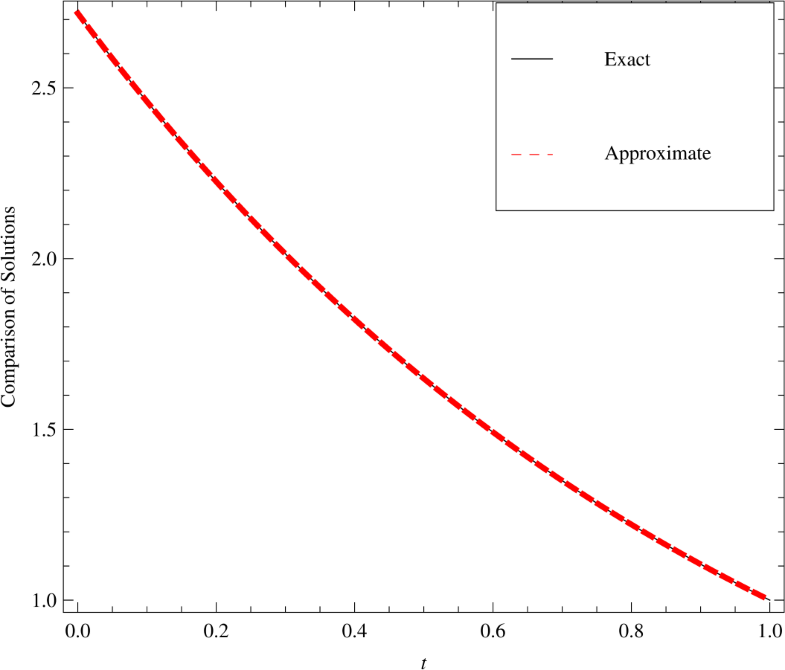Figure 2 Comparison of the 4th term MHATM solution and the exact solution of the TFNWS equation when $$x=1$$
To confirm the effectiveness and correctness of the MHATM for solving NWS equation, absolute error curves are given in Figs. 35. All figures show that our method converges quickly to the original solution only at the 4th-order approximation. Figures 35 illustrate that for $$\hbar =-1$$, the convergence is optimal.Figure 3 Plot of the absolute error of TFNWS equation when $$\hbar =-1$$. (a) $$E_{4}(\eta )= \vert \eta (x,t)-\eta _{4}(x,t) \vert$$. (b) The corresponding $$E_{4}(\eta )$$ when $$t=1$$Figure 4 Plot of the absolute error of TFNWS equation when $$\hbar =-1.2$$. (a) $$E_{4}(\eta )= \vert \eta (x,t)-\eta _{4}(x,t) \vert$$. (b) The corresponding $$E_{4}(\eta )$$ when $$t=1$$Figure 5 Plot of the absolute error of TFNWS equation when $$\hbar =-0.8$$. (a) $$E_{4}(\eta )= \vert \eta (x,t)-\eta _{4}(x,t) \vert$$. (b) The corresponding $$E_{4}(\eta )$$ when $$t=1$$
Figure 6 reflects the performance of the estimated solution $$\xi _{\mathrm{app}}(\eta ,t)$$. Here we find that solution gradually decreases when $$\eta =1$$ and $$\hbar =-1$$.Figure 6 Plot of $$\eta _{4}(x,t)$$ vs. time t at $$x=1$$ and different values of β
Table 1

$$L_{2}$$ and $$L_{\infty }$$ error norm when $$\beta =1$$

x

$$L_{2}$$ error norm

$$L_{\infty }$$ error norm

0.1

1.90588 × 10−14

4.55191 × 10−15

0.2

1.47661 × 10−14

5.66214 × 10−15

0.3

7.91959 × 10−15

8.88178 × 10−15

Table 2

$$L_{2}$$ and $$L_{\infty }$$ error norm when $$\beta =1$$

λ

$$L_{2}$$ error norm

$$L_{\infty }$$ error norm

0.1

2.67902 × 10−6

7.07209 × 10−6

0.2

2.51277 × 10−6

7.67880 × 10−6

0.3

2.93262 × 10−7

7.75736 × 10−7

Figure 7 shows the ħ-curve of TFNSW Eq. (1.1) for different values of λ. We see that the adequate range of ħ is $$-1.9\leq \hbar <0$$.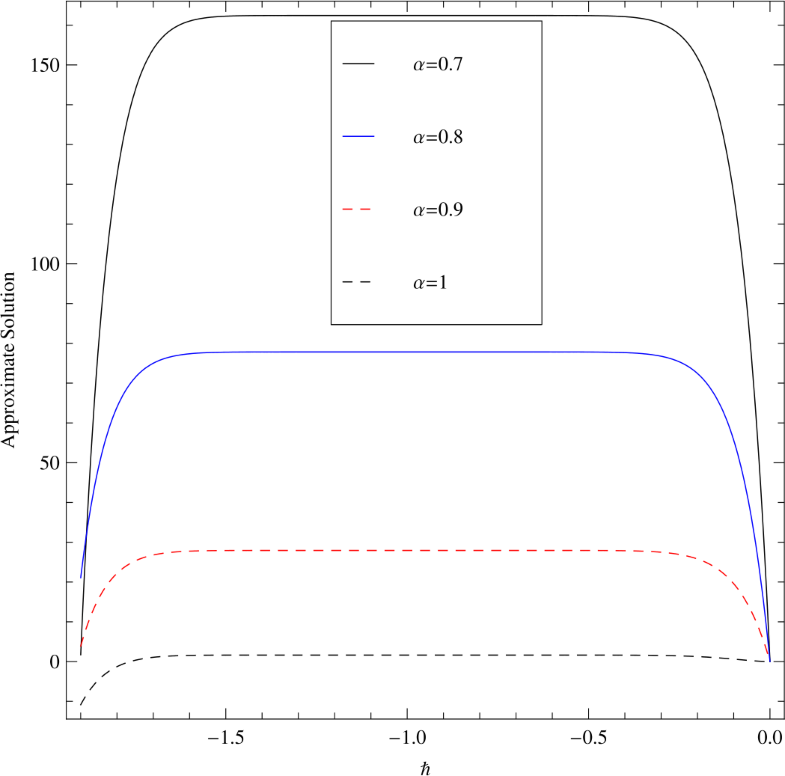Figure 7 Plot of ħ-curve for different values of β
Figure 8 shows the two-dimensional assessment among the exact and estimated solutions obtained by MHATM. At the same time, Figs. 9, 10, and 11 show the absolute error curve for $$\hbar =-1$$, $$\hbar =-1.2$$, and $$\hbar =-0.8$$, respectively.Figure 8 Comparison of the 4th term MHATM solution and the exact solution of the TFNWS equation when $$\lambda =1$$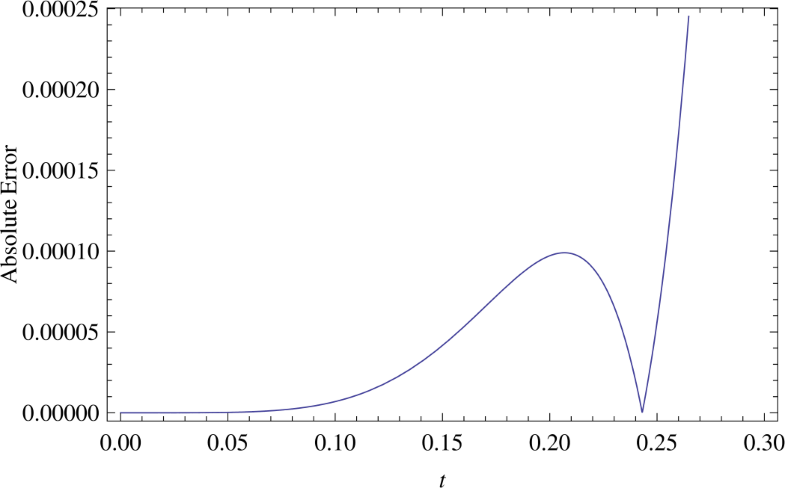Figure 9 Plot of the absolute error of TFNWS equation when $$\hbar =-1$$ and $$\lambda =1$$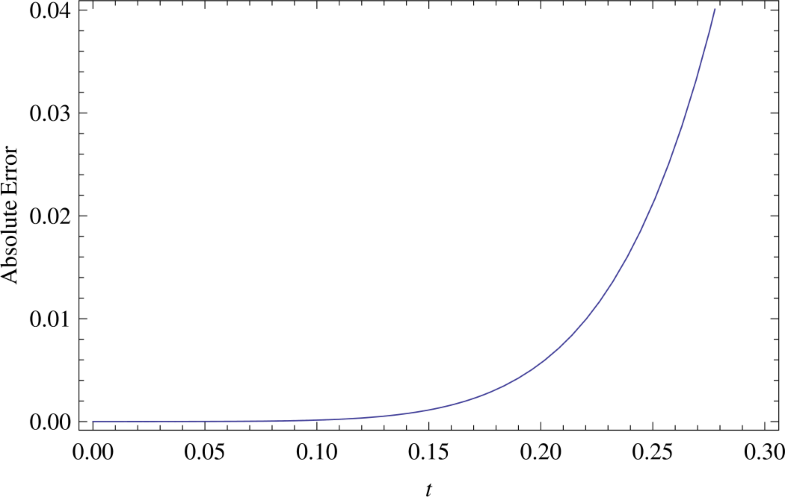Figure 10 Plot of the absolute error of TFNWS equation when $$\hbar =-1.2$$ and $$\lambda =1$$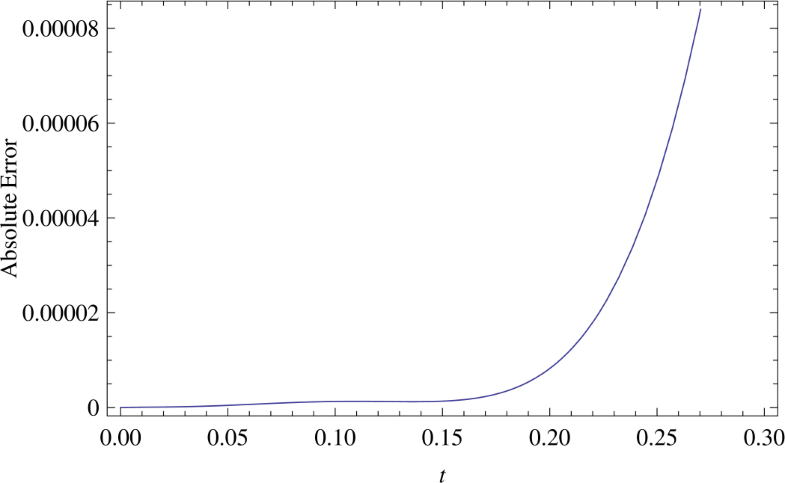Figure 11 Plot of the absolute error of TFNWS equation when $$\hbar =-0.8$$ and $$\lambda =1$$
Figure 12 reflects the performance of the estimated solution $$\xi (\eta ,t)$$ for different values of λ.Figure 12 Plot of $$\eta _{4}(x,t)$$ vs. time t at $$x=1$$ and different value of β
Nexture, in Fig. 13, the ħ-curve is given, which is also known as converging manage parameter. In this case, we can select that parameter in the range of $$-1.6< \hbar < -0.4$$.Figure 13 Plot of ħ-curve for different values of β

4.1 Comparison study

In this subsection, we discuss the comparison between the results obtained by MHATM and RPSM. Figure 14 shows for comparison of the results of Example 1, whereas Fig. 15 shows comparison of results of Example 2. From Figs. 1415 we can see that the solutions obtained by the MHATM and RPSM methods coincide with the exact solution, and both methods are consistent and efficient for solving fractional NSW equations.Figure 14 The surfaces show (a) the numerical approximate solution of $$\eta _{4}(x,t)$$ by MHATM when $$\lambda =1$$, (b) the numerical approximate solution of $$\eta _{4}(x,t)$$ by RPSM when $$\lambda =1$$, (c) the exact solution $$\eta (x,t)$$ when $$\lambda =1$$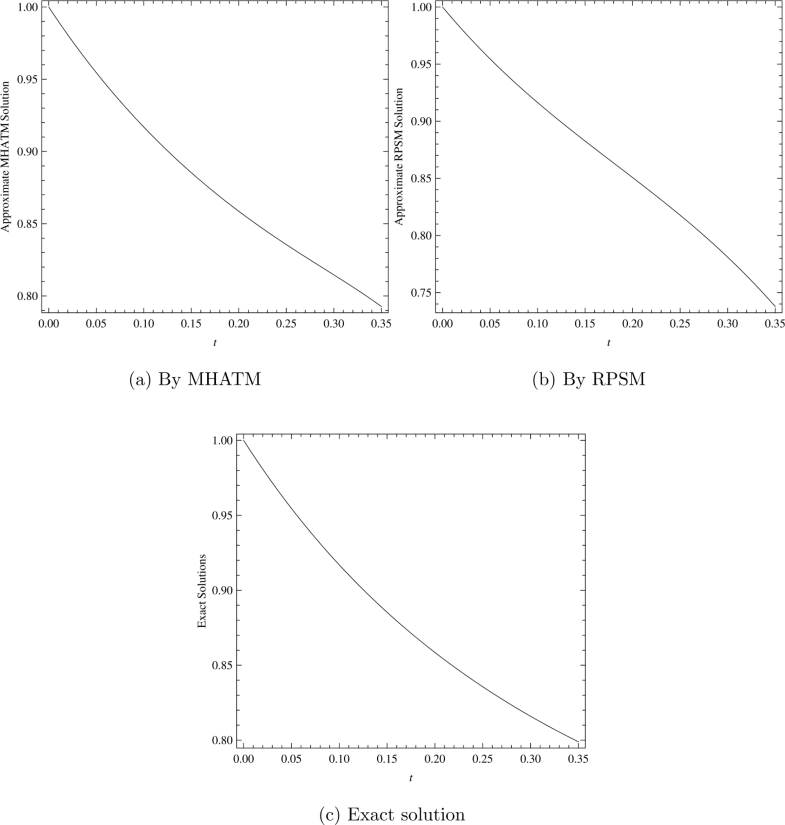Figure 15 The surfaces show (a) the numerical approximate solution of $$\eta _{4}(x,t)$$ by MHATM when $$\lambda =1$$, (b) the numerical approximate solution of $$\eta _{4}(x,t)$$ by RPSM when $$\lambda =1$$, (c) the exact solution $$\eta (x,t)$$ when $$\lambda =1$$

5 Concluding remarks

In this study, we proposed two powerful analytical methods for the solution of fractional Newell–Whitehead–Segel equations, which have the potential applications in bioengineering. To obtain the estimated solutions of the time-fractional Newell–Whitehead–Segel equations, we successfully applied the MHATM and RPSM. The accuracy and efficiency of the MHATM and RPSM are explained by examples. Moreover, the convergence analysis of both methods is discussed in detail. The results obtained for MHATM and RPSM are compared and plotted. From the figures we observe that the solution obtained by the MHATM and RPSM methods coincide with the exact solution, and both methods are consistent and efficient for solving fractional Newell–Whitehead–Segal equations. The fast convergence to the exact solutions of MHATM and RPSM shows that these methods are very suitable to solve FDEs. We conclude that the MHATM and RPSM methods are very effective and accurate techniques in the field of fractional-order differential equations. As a future work, we can implement the HAM to estimate the analytical solutions of fractional partial differential equations arising in engineering science by replacing the Laplace transform by a natural transform and the Caputo fractional operator by new fractional operators.

References

1. 1.
Podlubny, I.: Fractional Differential Equations. Academic Press, New York (1999)
2. 2.
Miller, K.S., Ross, B.: An Introduction to the Fractional Calculus and Fractional, Differential Equations. Willey, New York (1993)
3. 3.
Whitham, G.B.: Variational methods and applications to water wave. Proc. R. Soc. Lond. Ser. A 299(6), 6–25 (1967)
4. 4.
Kilbas, A.A., Srivastava, H.M., Trujillo, J.J.: Theory and Applications of Fractional Differential Equations. Elsevier, Amsterdam (2006)
5. 5.
Kumar, S.: Numerical computation of time-fractional Fokker–Planck equation arising in solid state physics and circuit theory. Z. Naturforsch. A 68, 777–784 (2013) Google Scholar
6. 6.
Kumar, S.: A new analytical modeling for telegraph equation via Laplace transforms. Appl. Math. Model. 38, 3154–3163 (2014)
7. 7.
Abbasbandy, S.: The application of homotopy analysis method to solve a generalized Hirota–Satsuma coupled KdV equation. Phys. Lett. A 361, 478–483 (2007)
8. 8.
Arqub, O.A., Al-Smadi, M.H.: Atangana–Baleanu fractional approach to the solutions of Bagley–Torvik and Painlevé equations in Hilbert space. Chaos Solitons Fractals 117, 161–167 (2018)
9. 9.
Arqub, O.A., Maayah, B.: Numerical solutions of integrodifferential equations of Fredholm operator type in the sense of the Atangana–Baleanu fractional operator. Chaos Solitons Fractals 117, 117–124 (2018)
10. 10.
Arqub, O.A.: Solutions of time-fractional Tricomi and Keldysh equations of Dirichlet functions types in Hilbert space. Numer. Methods Partial Differ. Equ. 34, 1759–1780 (2018)
11. 11.
Arqub, O.A.: Numerical solutions for the Robin time-fractional partial differential equations of heat and fluid flows based on the reproducing kernel algorithm. Int. J. Numer. Methods Heat Fluid Flow 28, 828–856 (2018) Google Scholar
12. 12.
Muhammed, F.B., Silambarasan, R., Zakia, H., Mekkaoui, T.: New and extended applications of the natural and Sumudu transforms: fractional diffusion and Stokes fluid flow realms. In: Advances in Real and Complex Analysis with Applications, pp. 107–120. Birkhauser, Singapore (2017)
13. 13.
Zakia, H., Mekkaoui, T.: Approximate analytical and numerical solutions to fractional KPP-like equations. Gen 14, 1–9 (2013)
14. 14.
Zakia, H., Mekkaoui, T.: Approximate analytical solution to a time-fractional Zakharov–Kuznetsov equation. Intern. J. Phys. Research 1, 28–33 (2013) Google Scholar
15. 15.
Odibat, Z., Bataineh, A.S.: An adaptation of homotopy analysis method for reliable treatment of strongly nonlinear problems: construction of homotopy polynomials. Math. Methods Appl. Sci. 38(5), 991–1000 (2015)
16. 16.
Kumar, S., Rashidi, M.M.: New analytical method for gas dynamics equation arising in shock fronts. Comput. Phys. Commun. 185, 1947–1954 (2014)
17. 17.
Kumar, S., Kumar, A., Argyros, I.K.: A new analysis for the Keller–Segel model of fractional order. Numer. Algorithms 75(1), 213–228 (2017)
18. 18.
Kumar, A., Kumar, S.: A modified analytical approach for fractional discrete KdV equations arising in particle vibrations. Proc. Natl. Acad. Sci. India Sect. A Phys. Sci. 8(1), 95–106 (2018)
19. 19.
El-Ajou, A., Arquba, O.A., Momani, S.: Approximate analytical solution of the nonlinear fractional KdV–Burgers equation: a new iterative algorithm. J. Comput. Phys. 293, 81–95 (2015)
20. 20.
Kumar, S., Kumar, A., Baleanu, D.: Two analytical methods for time-fractional non-linear coupled Boussinesq–Burgers equations arise in propagation of shallow water waves. Nonlinear Dyn. 85(2), 699–715 (2016)
21. 21.
Yao, J.J., Kumar, A., Kumar, S.: A fractional model to describe the Brownian motion of particles and its analytical solution. Adv. Mech. Eng. 7(12), 1–11 (2015) Google Scholar
22. 22.
Momani, S., Abu Arqub, O., Hammad, M.A., Hammour, Z.A.: A residual power series technique for solving systems of initial value problems. Appl. Math. Inf. Sci. 10, 765–775 (2016) Google Scholar
23. 23.
Kumar, A., Kumar, S., Yan, S.P.: Residual power series method for fractional diffusion equations. Fundam. Inform. 151(1–4), 213–230 (2017)
24. 24.
Kumar, A., Kumar, S.: Residual power series method for fractional Burger types equations. Nonlinear Eng. 5(4), 235–244 (2016)
25. 25.
Prakash, A., Verma, V.: Numerical method for fractional model of Newell–Whitehead–Segel equation. Front. Phys. 7, 15 (2019) Google Scholar
26. 26.
Saberi, E., Hejazi, S.R., Motamednezhad, A.: Lie symmetry analysis, conservation laws and similarity reductions of Newell–Whitehead–Segel equation of fractional order. J. Geom. Phys. 135, 116–128 (2019)
27. 27.
Rao, A.M., Warke, A.S.: Approximate and analytic solution of some nonlinear diffusive equations. In: Advances in Mathematical Methods and High Performance Computing, pp. 487–499. Springer, Cham (2019) Google Scholar
28. 28.
Korkmaz, A.: Complex wave solutions to mathematical biology models I: Newell–Whitehead–Segel and Zeldovich equations. J. Comput. Nonlinear Dyn. 13(8), 081004 (2018) Google Scholar
29. 29.
Patade, J., Bhalekar, S.: Approximate analytical solutions of Newell–Whitehead–Segel equation using a new iterative method. World J. Model. Simul. 11(2), 94–103 (2015) Google Scholar
30. 30.
Saravanan, A., Magesh, N.: A comparison between the reduced differential transform method and the Adomian decomposition method for the Newell–Whitehead–Segel equation. J. Egypt. Math. Soc. 21(3), 259–265 (2013)
31. 31.
Mangoub, M.M., Sedeeg, A.K.: On the solution of Newell–Whitehead–Segel equation. Am. J. Math. Comput. Model. 1(1), 21–24 (2016) Google Scholar
32. 32.
Baishya, C.: Solution of Newell–Whitehead–Segel equation with power law nonlinearity and time dependent coefficients. Int. J. Adv. Appl. Math. Mech. 3(2), 59–64 (2015)
33. 33.
Nourazar, S.S., Soori, M., Nazari-Golshan, A.: On the exact solution of Newell–Whitehead–Segel equation using the homotopy perturbation method (2015) arXiv preprint. arXiv:1502.08016
34. 34.
Macías-Díaz, J.E., Ruiz-Ramírez, J.: A non-standard symmetry-preserving method to compute bounded solutions of a generalized Newell–Whitehead–Segel equation. Appl. Numer. Math. 61(4), 630–640 (2011)
35. 35.
Vaneeva, O., Boyko, V., Zhalij, A., Sophocleous, C.: Classification of reduction operators and exact solutions of variable coefficient Newell–Whitehead–Segel equations. J. Math. Anal. Appl. 474, 264–275 (2019)
36. 36.
Ahmad, J.: The modified variational iteration method on the Newell–Whitehead–Segel equation using He’s polynomials. Math. Theory Model. 7, 11 (2017) Google Scholar
37. 37.
Prakash, A., Kumar, M.: He’s variational iteration method for the solution of nonlinear Newell–Whitehead–Segel equation. J. Appl. Anal. Comput. 6(3), 738–748 (2016)
38. 38.
Graham, R.: Systematic derivation of a rotationally covariant extension of the two-dimensional Newell–Whitehead–Segel equation. Phys. Rev. Lett. 76(12), 2185 (1996)
39. 39.
Graham, R.: Erratum: systematic derivation of a rotationally covariant extension of the two-dimensional Newell–Whitehead–Segel equation [Phys. Rev. Lett. 76, 2185 (1996)]. Phys. Rev. Lett. 80(17), 3888 (1998) Google Scholar
40. 40.
Kumar, D., Sharma, R.P.: Numerical approximation of Newell–Whitehead–Segel equation of fractional order. Nonlinear Eng. 5(2), 81–86 (2016) Google Scholar
41. 41.
Nourazar, S.S., Parsa, H., Sanjari, A.: A comparison between Fourier transform Adomian decomposition method and homotopy perturbation method for linear and non-linear Newell–Whitehead–Segel equations. AUT J. Model. Simul. 49(2), 227–238 (2017) Google Scholar
42. 42.
Rojas, R.G., Elías, R.G., Clerc, M.G.: Dynamics of an interface connecting a stripe pattern and a uniform state: amended Newell–Whitehead–Segel equation. Int. J. Bifurc. Chaos 19(08) 2801-12 (2009)
43. 43.
Valls, C.: Algebraic traveling waves for the generalized Newell–Whitehead–Segel equation. Nonlinear Anal., Real World Appl. 36, 249–266 (2017)
44. 44.
Prakash, A., Goyal, M., Gupta, S.: Fractional variational iteration method for solving time-fractional Newell–Whitehead–Segel equation. Nonlinear Eng. 8(1), 164–171 (2018) Google Scholar
45. 45.
Edeki, S.O., Ogundile, O.P., Osoba, B., Adeyemi, G.A., Egara, F.O., Ejoh, A.S.: Coupled FCT-HP for analytical solutions of the generalized time fractional Newell–Whitehead–Segel equation. WSEAS Trans. Syst. Control 13, 266–274 (2018) Google Scholar
46. 46.
Aasaraai, A.: Analytical solution of the Newell–Whitehead–Segel equation by differential transform method. Middle-East J. Sci. Res. 10(2), 270–273 (2011)
47. 47.
Nourazar, S.S., Soori, M., Golshan, A.N.: On the exact solution of Newell–Whitehead–Segel equation using the homotopy perturbation method. Aust. J. Basic Appl. Sci. 5(8), 1400–1411 (2011) Google Scholar
48. 48.
Malomed, B.A.: Stability and grain boundaries in the dispersive Newell–Whitehead–Segel equation. Phys. Scr. 57, 115–117 (1998) Google Scholar
49. 49.
Pue-on, P.: Laplace Adomian decomposition method for solving Newell–Whitehead–Segel equation. Appl. Math. Sci. 7, 6593–6600 (2013)
50. 50.
Liao, S.J.: The proposed homotopy analysis technique for the solution of nonlinear problems. PhD thesis, Shanghai Jiao Tong University (1992) Google Scholar

© The Author(s) 2019

Authors and Affiliations

• Sunil Kumar
• 1
• Amit Kumar
• 2
• Shaher Momani
• 3
• 4
• Mujahed Aldhaifallah
• 5
• Kottakkaran Sooppy Nisar
• 6
Email author
1. 1.Department of MathematicsNational Institute of TechnologyJamshedpurIndia
2. 2.Department of MathematicsBalarampur College PuruliaWest BengalIndia
3. 3.Department of Mathematics and Sciences, College of Humanities and SciencesAjman UniversityAjmanUAE
4. 4.Department of Mathematics, Faculty of ScienceUniversity of JordanAmmanJordan
5. 5.Systems Engineering DepartmentKing Fahd University of Petroleum and MineralsDhahranSaudi Arabia
6. 6.Department of Mathematics, College of Arts and SciencesPrince Sattam Bin Abdulaziz UniversityWadi AldawasirKingdom of Saudi Arabia

Personalised recommendations